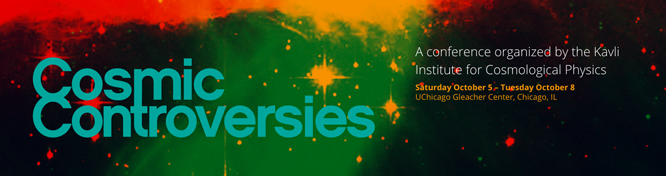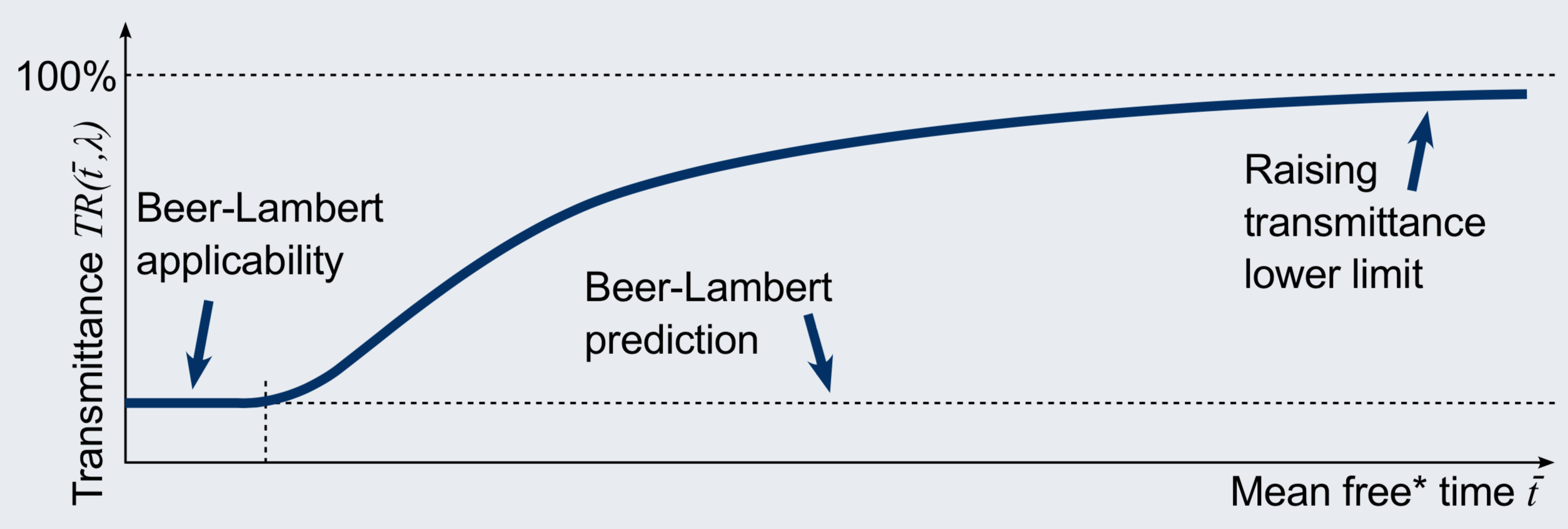# The Theory

The full theory is described in the articleThe overview presented below is based on the poster prepared for the Cosmic Controversies 2019 conference### Background

##### Dark Matter & non-locality
• It is generally believed that Dark Matter can’t be baryonic gas (H, He) or dust because it would be opaque. This is in accordance with the classic Beer-Lambert law (and it derivatives) that relates gas transmittance to optical path length $l$, concentration $c$ and molar absorptivity $\epsilon(\lambda)$ only, assuming molecules locality: $TR(\lambda)=10^{-lc\varepsilon(\lambda) }$
• Increasing number of experiments show non-local matter properties, e.g. quantum entanglement, Bose-Einstein condensate, helium Young’s type double-slit experiment, etc

### Hypothesis

##### New transmittance model is required
• The classic Beer-Lambert law is not applicable to interstellar medium
• We should take into account a solution of the Schrödinger equation for a free particle for each gas molecule for ultra diluted gas
• Wavefunctions spreading & detector size affects optical transmittance measurement

### Methods

##### Non-locality applied for transmittance model
• Model a non relativistic gas molecule as a gaussian wave packet
• A molecule is free between consecutive collisions with either *photon or *molecule: mean free* time $\overline{t}$
• Molecules’ wavefunctions spread according to the Schrödinger equation for a free particle, it is smeared gas
• Assume a wavelenght dependend cross section $\sigma(\lambda)$ remains constant on spreading, i.e. constant Einstein coefficients
• If a detector area $A$ is smaller than molecule spread, a photon scattering out of detectability tunnel $T$ can’t be observed, probability of possibly observed scattering event reads as:$$P^{obs}\left ( \overline{t},\lambda \right )=C(\sigma(\lambda), \sigma_A, geometry)\int_T\left | \mathit{\Psi} (\boldsymbol{r}, \overline{t} ) \right |^2dr ,$$ where $C$ depends on geometry of actual setup, total cross-section and detector dimensions and efficiency
• Taking $N$ independent molecules, where incident photon may be scattered by any of them, a Markov chain is used for the transmittance equation:$$TR ( \overline{t},\lambda )=\prod_{n=1}^N\left ( 1-P_n^{obs} ( \overline{t},\lambda ) \right )$$
• Analytical form of the transmittance lower limit may be presented

### Results

##### Measured gas transmittance may raise to 100%Smeared gas Transmittance vs Mean free* time \overline{t} for \Psi as gaussian wave packet and square detector A
• Transmittance of smeared gas depends also on a detector size and mean free* time $\overline{t}$
• Raising mean free* time $\overline{t}$ leads to raising gas transmittance measured with a small detector:

$TR ( \overline{t},\lambda )\geqslant \prod_{n=1}^N\left [ 1- \frac{C(...)}{4} \left ( erf \left( \frac{dist_n-\sqrt{A}}{\sqrt2 stdev(\bar{t})} \right) \newline - erf \left( \frac{dist_n+\sqrt{A}}{\sqrt2 stdev(\bar{t})} \right) \right )^2 \right ]$

where $\Delta$-initial position measurement accuracy, $m$-molecule mass, $dist_n$- distance from $\left \langle x_n \right \rangle$ to tunnel $T$ axis and $$stdev(\bar{t})=\sqrt{\frac12 \left( \Delta^2+\frac{\hbar^2\bar{t}^2}{m^2\Delta^2} \right)}$$
• Transmittance smaller as detector gets bigger
• Interstellar medium transmittance depends on background radiation intensity
• Calculations confirm intragalactic radiation is so weak thay neutral atomic hydrogen (HI) may form smeared gas clouds
• It is shown, the classic Beer-Lambert law is the first-order approximation of the proposed model

### Conclusions

##### Model is falsifiable, the ideal candidate for Dark matter
• Achieved results interesting for QM measurement & decohorence discussions
• A variety of possible laboratory or orbital experiments for detecting smeared gas are proposed in the paper
• Geocorona may be smeared (H) gas as its observed „density” depends on Earth shadow & Sun activity
• Baryonic (H, He) Dark Matter would be ultra diluted gas, intragalactic radiation is weak enough so clouds of smeared gas may be a component of Dark matter